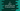# C program to print the current hour, minute and second values## C program to print the current hour, minute and second values:

In this post, we will learn how to print the current hour, minute and second values in the console. For dealing with time, C provides one header file time.h. It provides couple of different time related functions, four variable types and two Macros.

In this post, we will learn how to print the current hour, minute and second values of current time in C.

### Functions we need to use:

Below are the functions of time.h that we need to use to print the current time values:

#### Function 1:

``time_t time(time_t *t)``

It returns the time in time_t format. Actually, it returns the Epoch time or time in seconds since UTC, January 1, 1970. We can pass the parameter as NULL to this function to get the Epoch time. This is the first function that we need to call.

Then, we need to call the localtime() function which is defined as below:

#### Function 2:

``struct tm *localtime(const time_t *t)``

This function takes one time_t value as argument and returns one structure of type tm that holds different time related values. For printing the hour, minute and second values, we can use tm_hour, tm_min and tm_sec properties of tm structure.

### Complete C program:

Below is the complete c program :

``````#include <stdio.h>
#include <time.h>

int main()
{
time_t seconds;
struct tm *timeStruct;

seconds = time(NULL);

timeStruct = localtime(&seconds);

printf("Current time : %d:%d:%d\n", timeStruct->tm_hour, timeStruct->tm_min, timeStruct->tm_sec);
}``````

Here,

• seconds is a variable of type time_t.
• timeStruct is a tm structure.
• Using time function, we are getting the value of seconds and using localtime, we are getting timeStruct.
• Finally, we are printing the current hour, minute, seconds from the structure timeStruct.

Running this program will print one output something like below :

``Current time : 15:59:39``

Based on the system time, we will get the hour, minute and seconds values.